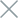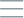• Home
• Tags
• Series
•# Python Syntax: Variables, Data Types, Conditionals, Loops

## Variables and Data Types

Python is a dynamically typed programming language, meaning you do not need to declare variable types before using them. Below are examples of variable declaration and some common data types:

Variable declaration:

``````variable_name = value
``````

Common data types:

• Integer (`int`): `age = 25`
• Floating-point number (`float`): `pi = 3.14`
• String (`str`): `name = "John"`
• Boolean (`bool`): `is_true = True`

## Conditional Statements

Conditional statements in Python are used to check conditions and execute statements based on the evaluation result. The `if`, `else`, and `elif` (else if) structures are used as follows:

`if` statement:

``````if condition:
# Execute this block if condition is True
``````

`else` statement:

``````else:
# Execute this block if no preceding if statement is True
``````

`elif` `(else if`) statement:

``````elif condition:
# Execute this block if condition is True and no preceding if or else statement is True
``````

## Loops

Python supports two commonly used loop types: `for` loop and `while` loop, enabling repetitive execution of statements.

`for` loop:

``````for variable in sequence:
# Execute statements for each value in the sequence
``````

`while` loop:

``````while condition:
# Execute statements while the condition is True
``````

Specific Example:

``````# Variable declaration
age = 25
name = "John"

# Conditional statement
if age >= 18:
print("You are of legal age.")
else:
print("You are not of legal age.")

# Loop
for i in range(5):
print("Hello there!")

count = 0
while count < 5:
print("Loop number:", count)
count += 1
``````

When executed, the above code will check the age and print the appropriate message, then loop the `Hello there!` message five times using a `for` loop, and finally print the values of the `while` loop.